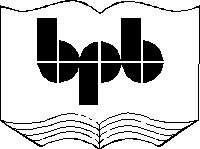10

Miscellaneous

This is the final chapter of the book and contains a medley of a vast amount of miscellaneous information that could not be classified into any of the earlier chapters. It covers a vast of topics that include creation and initialization of variables, the lifetime and scope of variables, the concept of a namespace, the mechanism for displaying special characters, the concept of unicode, the break and continue statements, the function of operators such as the comma operator, the NOT operator, the + operator, the ++ opeartor, the ? : construct , the difference between = and = =, precedence of operators and error checking. Without further ado, we shall go right ahead with the explanations since we have a lot of ground to cover.

When you create a variable, you can initialize it at the same time. You can subsequently change the value of the variable, but you cannot create it yet again in the same program.

aaa.wmls

extern function abc()

{

var i = 10;

i = i + 10;

var i = "no";

}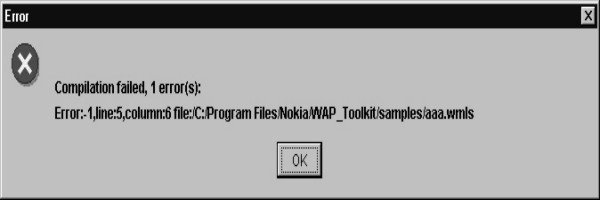Screen 10. 1

In the above example, line 3 will generate an error because the variable i has been declared again.

You can create a new variable anywhere in the program in WMLScript. You don't need to create all the variables at the beginning of the program, as some programming languages like C compel you to.

The next example shows that you can create a variable in an if statement also. Here, the program creates a variable z within the if statement. However, an error is generated when the program tries to create the variable z again in the else statement also, because the same variable cannot be created twice.

aaa.wmls

extern function abc()

{

var aa;

var i = 10;

if ( i >=5 )

var z = 10;

else

var z = 20;

}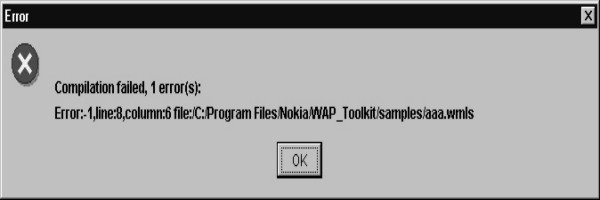Screen 10. 2

Logically, either the if statement will get called or the else statement gets called. Therefore, the variable will get created only once. But, this logic is not applied by the compiler  which  generates an error.

The next example shows that in the if statement, the variables y and z are created. If you create a variable and do not initialise it with a value, its value will be blank by default. If you pass this uninitialised variable as a parameter to the function String.format, the function will also consider its value to be blank by default. But in this case, you would have realised that the variable z doesn't get created at all because the else statement does not get executed at all. Even then, no error is generated when the String.format function is called. Therefore, the Dialogs.alert does not get called.

aaa.wmls

extern function abc()

{

var aa;

var i = 10;

if ( i >=5 )

var y = 10;

else

var z = 20;

aa = String.format("%d..",z);

}

The variable y does not create any problem. Since, the else statement does not get called, the variable z doesn't get created at all and hence, it has an invalid value. If the variable z had been created and not initialized, it would have had NULL value.

You can create variables anywhere in the program. Furthermore, the variable is visible or available to the program, from the place from which it has been created. This rule has the following repercussions:

Variables aa and i are available to the if statement and beyond.

Variable z is available to the else statement and beyond.

The variables aa, i, y and z are available to the String.format function.

So, to sum up,

· If you create a variable and don't initialize it, its value will be NULL.

· If you use this uninitialised variable, its value will be invalid.

· You can create a variable wherever you want, in the program. However, it is preferable to create it  initially, at the top.

· The scope or the lifetime of a variable is from the point it is created, to the end of the program.

The following program is very elementary. Here, we have initialized aa and bb to the value of 20.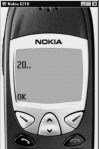Screen 10. 3

aaa.wmls

extern function abc()

{

var aa,bb,cc;

aa = 10;

bb = 20;

aa = bb;

bb = 30;

cc = String.format("%d..",aa);

}

When the statement aa=bb is executed, the values of aa and bb are 20 each. Thereafter, when the value of bb is changed to 30, it does not alter the value of the variable aa. There is no link between aa and bb at this stage.

In the following example, we have a WML file where we have initialized the variable aa to "hell".

a11.wml

<?xml version="1.0"?>

<!DOCTYPE wml PUBLIC "-//WAPFORUM//DTD WML 1.1//EN" "http://www.wapforum.org/DTD/wml_1.1.xml">

<wml>

<card newcontext="true">

<do type="xx">

<go href="aaa.wmls#abc()">

<setvar name="aa" value ="hell"/>

</go>

</do>

<p>

Hello \$aa

</p>

</card>

</wml>

We are displaying "Hello \$aa". Click on Options and start rolling.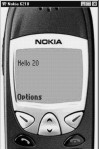Screen 10. 4

aaa.wmls

extern function abc()

{

var aa = 10;

WMLBrowser.setVar("aa","20");

aa = 30;

WMLBrowser.refresh();

}

We have created a variable called aa and then using the function setVar, we have initialised the value of aa to 20. Thereafter, the program once again initializes the variable aa to 30. What we are trying to demonstrate here is that the variable aa in WMLScript is different from the variable aa of WML. The only way you can change the variable aa of WMLScript is by using setVar.

If you remove this WMLBrowser, and initialize aa to 10, the value of aa would remain as "hell". Only when you use setVar, the value of aa becomes 20. This means that the variable aa in WMLScript has nothing in common with the variable aa  in WML.

Calling a Function From compiled WMLScript File

So far, we have learnt how to call a function from WML, and how to call one function from another function. Now, we shall see how to call a function from another WMLScript file.

aaa.wmls

use url zzz "file:/C:/Program Files/Nokia/Nokia WAP

Toolkit/samples/a111.wmlsc";

extern function abc() {

zzz#pqr();

}

a111.wmls

extern function pqr()

{

}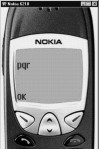Screen 10. 5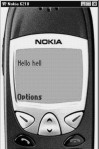Screen 10. 6

The syntax use is called a pragma. You can have different texts after use. Here we have used url. You can also use words like access. Thereafter, we have used the text zzz, which is just a name. The zzz represents a url in this example. A url is the name of a compilation unit. Remember, we cannot say wmls because what it requires is a compiled version of the script and not the text version.

Next, we have used the word file because that's the location from where we want the requisite information. We could have used http:// if we wanted to obtain the information from the Internet. In order to call a function from the WMLScript file a111.wmls, you can simply use zzz# followed by pqr. Do not forget to compile the file a111.wmls.

What is a namespace ?

A namespace has a specific connotation in WMLScript. A namespace is the nomenclature for a specific area in memory. Variables and functions have names. WMLScript stores these names in different parts of memory, each of which has a different namespace. Due to this, we say that each of these variables or functions has a different namespace. A function, a variable and a pragma can have the same name because these names are stored in different parts of memory, i.e. they have different namespaces. But, the names of parameters, functions and variables cannot be the same because they have a common namespace.

a11.wml

<?xml version="1.0"?>

<!DOCTYPE wml PUBLIC "-//WAPFORUM//DTD WML 1.1//EN" "http://www.wapforum.org/DTD/wml_1.1.xml">

<wml>

<card newcontext="true">

<do type="xx">

<go href="aaa.wmls#zzz()">

<setvar name="aa" value ="hell"/>

</go>

</do>

<p>

Hello \$aa

</p>

</card>

</wml>

aaa.wmls

use url zzz "http://toolkit/C:/Progra~1/Nokia/WAP_Toolkit/samples/a111.wmlsc";

extern function zzz() {

var zzz = 10;

zzz#zzz(zzz);

}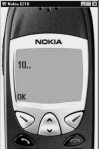Screen 10. 7

a111.wmlsc

extern function zzz(aaa)

{

var bbb ;

bbb = String.format("%d..",aaa);

}

In case you don’t see the output as we shown you, select the Messages tab and check the Detail.option. You wiill see the url that Nokia frames to pick up the files. Add the same url in doublequotes on the first line. Note the file is a111.wmlsc.

In the above example, we have a pragma called zzz, a function called zzz and a variable called zzz. This does not generate any error as this is valid, since they all have different namespaces.

In the following program, we have a variable j in the for loop. Everytime the for loop does an iteration, the variable j gets created and initialized to the value of 20.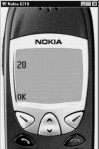Screen 10. 8

aaa.wmls

extern function abc() {

var i,aa;

for(i=0;i<=5;i++)

{

var j=20;

aa=String.format("%d",j);

}

}

If you want to display certain special characters, you have to prefix them with a backslash. Some of these special characters are as follows:

\\ - To display a backslash.

\n - Represents a new line.

\b - Represents a backspace

\t  - Represents a tab.

You should try it out in other user agents, since all of them don't work in Nokia.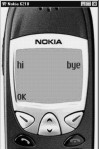Screen 10. 9

aaa.wmls

extern function abc()

{

}

If you want to represent a number in hexadecimal value, you have to prefix it with \x. If you want to represent it in ASCII value, you have to use its ASCII value.

For example,

· The hexadecimal value of the character A is \x41.

· The ASCII value of the character A is 65.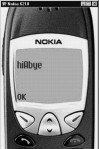Screen 10. 10

aaa.wmls

extern function abc()

{

}

If you want to represent a character in unicode, you have to prefix it with \u00. It is similar to the hexadecimal format, but instead of an 8 bit number, it uses a 16 bit number.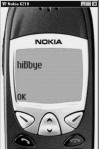Screen 10. 11

aaa.wmls

extern function abc()

{

}

Unicode

What is meant by unicode? It is a standard that the computer industry came up with, so that they could ensure that all languages in the world could be expressed on a computer. There are certain languages like Chinese and Japanese that are very visual in nature and have a very large character set. ASCII is adequate in expressing the letters of the English alphabet, but not good enough for express these visual languages. Thus, the unicode standard was established to enable the characters of these visual languages to be represented on the computer screen.

break

There is a construct called break. It is used to break out of a for loop or a while loop. If you are within a loop and for some reason, you want to get out of the loop without waiting for the loop condition to evaluate to a false value, break can be used.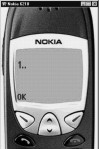Screen 10. 12

aaa.wmls

extern function abc()

{

var z,aa;

for ( z = 1 ; z <= 5 ; z++)

{

if ( z == 2)

break;

aa = String.format("%d..",z);

}

}

In the above example, when z reaches the value of 2, the break statement will get executed and the program will go out of the for loop. Normally, every for or while loop has an exit condition which when false, takes the program out of the loop. But if, for any reason, you don't want to wait for that condition to be false, you can use the break statement to prematurely break out of the loop. In the above example, since the program exits from the loop as soon as the value of z equals 2, you get to see the Dialogs.alert only once.

The reverse of the break statement is the continue statement. When a program reaches the continue statement in a for or a while loop, the program does not complete the rest of the for or while loop, but returns to  the beginning of the loop without executing any of the remaining statements of the loop.

aaa.wmls

extern function abc()

{

var z,aa;

for ( z = 1 ; z <= 5 ; z++)

{

if ( z == 2)

continue ;

aa = String.format("%d..",z);

}

}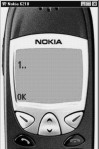Screen 10. 13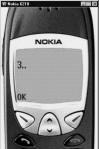Screen 10. 14

Initially, when the value of z is 1, the if statement evaluates to false and hence, the continue statement does not get executed. When the value of z becomes 2, the continue statement gets executed and takes the control back to the beginning of the for loop. As a result, the 2 lines following the continue statement, upto the closing brace of the for statement, don't get called. The output will be 1 3 4 5.

Thus, the break statement gets you out of a loop and a continue statement takes you back to the beginning of the loop.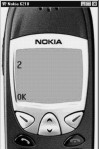Screen 10. 15

aaa.wmls

extern function abc()

{

var z,aa;

for ( z = 1 ; z <= 5 ; z++)

{

if ( z = 2)

break;

aa = String.format("%d..",z);

}

aa = String.format("%d",z);

}

There is a major difference between the equal to sign i.e. = ,and the double equal to sign i.e. = =.

· The = sign is used for initialisation of the value of a variable.

· The = = sign is used to verify whether the values on both sides of this symbol are equal in value or not.

In the above example, z is initialized to 1 at the start of the loop. The if statement checks whether the value of z is equal to 2 or not. Since it is not, the break statement does not get executed.

In the next iteration, the value of z becomes 2 and the if statement evaluates to true. Thus, the break statement gets executed and the program exits from the loop.

The program reaches the function String.format which displays the value of z as 2.

The + operator

In the context of strings, the + operator is used to concatenate strings. For example, if you use "hi"+"bye", the result will be "hibye".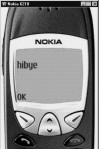Screen 10. 16

aaa.wmls

extern function abc()

{

}

The NOT sign is very useful because it inverts the value of the variable it acts upon; in other words, if the value of the variable is true, it makes it false, and if it is false, it makes it true.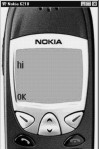Screen 10. 17

aaa.wmls

extern function abc()

{

if ( !false)

}

Where can you use the NOT condition ?

If you have a complex condition, and you want to make it easy for anyone who reads your program to understand the logic, you can put a NOT before the condition. This implies that, if the condition is false, then return true, and vice versa.

There are many programming constructs that are very verbose. For example, if there is a simple if and else construct, it stretches over 4 lines. To circumvent this, and to make the code more compact, there is a operator as follows :

condition ? statement1 : statement2

If the condition before the question mark is true, the statement1 gets executed; otherwise statement 2 gets executed. The C language also has this operator.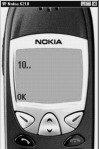Screen 10. 18

aaa.wmls

extern function abc()

{

var i;

var aa;

i = 3 > 1 ? 10 : 20 ;

aa = String.format("%d..",i);

}

In the for loop syntax, you can initialise variables by inserting them before the first semicolon and separating them with commas. This has been shown in the following example.

aaa.wmls

extern function abc()

{

var i,j,k,aa;

for ( i = 0, j= 0; i <= 5; i++,j++)

{

k = i + j;

aa = String.format("%d..",k);

}

}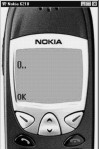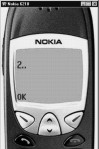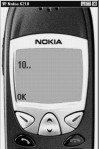Screen 10. 19 Screen 10. 20 Screen 10. 21

The comma Operator

It may be noted that we have separated the variables by commas in the for loop, while initialising and incrementing them. If you had not used the comma operator, you would have had to put the incrementing statements inside the for loop.

The other point to be  noted is that comma operator ensures that the last value which is provided, is the final value of the statement. Eg- If you use i=67,32, the resultant value of i will be 32 and not 67.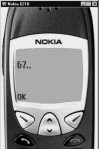Screen 10. 22

aaa.wmls

extern function abc()

{

var i,aa;

i = (67,32);

aa = String.format("%d..",i);

}

The following example is slighty more complicated, since we are calling a function called pqr .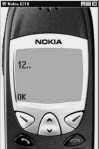Screen 10. 23

aaa.wmls

extern function abc()

{

var a = 2;

var b = 3;

var c = (a , 4);

pqr(3*(b*a,c));

}

extern function pqr(aa)

{

var bb;

bb = String.format("%d..",aa);

}

First, we are saying c=a,4. So, the resultant value of c is 4 due to the effect of the comma operator. Since, a bracket and a comma are present in the funtion pqr, the expression" b*a" doesn't get evaluated. What gets evaluated is c, whose value is 4. So, the resultant value that is passed to the function pqr is 4* 3 which is 12. Therefore, the value of aa that gets printed is 12.

The function isvalid decides whether a statement is valid or not.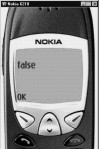Screen 10. 24

aaa.wmls

extern function abc()

{

var i;

i = isvalid 1/0 ;

if ( i )

else

}

You don't have to put brackets around the parameters of this function.

In the above example, 1/0 is infinity; so, it is invalid. The function isvalid therefore, return false.

If you put the parameter in double inverted commas, it becomes a string. In this case, the isvalid function will return true. If the invalid is passed an invalid value without the double inverted commas, it will return a false.

Now, invalid is a problematic because anything that is invalid, always remains invalid.

We are saying 1/0 && pqr.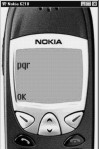Screen 10. 25

aaa.wmls

extern function abc()

{

1/0 && pqr();

}

extern function pqr()

{

}

In the above example, 1/0 is infinity and considered to be true. So, the pqr function gets called. If you prefix a number with 0x, it is interpreted as a hex number.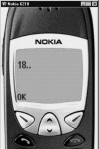Screen 10. 26

aaa.wmls

extern function abc()

{

var i,aa;

i = 0x12;

aa = String.format("%d..",i);

}

If you prefix a number with an 0, it is interpreted as an octal number. You can write a number either in its decimal form or in its octal form.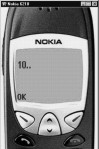Screen 10. 27

aaa.wmls

extern function abc()

{

var i,aa;

i = 012;

aa = String.format("%d..",i);

}

The next example shows that if you don't initialize a variable, it will contain an empty string. Thus, if you use it anywhere, it will not have any effect. Therefore, since the variable aa is an empty string in the example below,  the resultant string is "hibye", which is without any space between "hi" and "bye".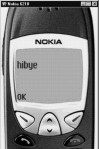Screen 10. 28

aaa.wmls

extern function abc()

{

var aa;

}

The div operator

The expression 3/2 gives the answer 1.5  and  3 div 2 gives the answer 1 because the result of the div operator is always an integer value. The div operator is used whenever you want to divide one number by another, and you are interested only in the quotient and not in the remainder.

aaa.wmls

extern function abc()

{

var aa;

aa = String.format("%f..",3/2);

aa = String.format("%f..",3 div 2);

}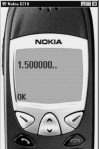Screen 10. 29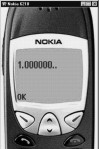Screen 10. 30

The % operator

At times, you may only want the remainder and not the quotient. In such a case, you can use the % operator. The % operator gives you MODULUS of the division, i.e. it returns the value of the remainder. In the following example, the resultant value of 6 % 4 is 2.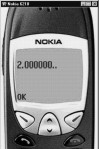Screen 10. 31

aaa.wmls

extern function abc()

{

var aa;

aa = String.format("%f..", 6 % 4);

}

In the following example, 6 > 4 is false. Hence, its resultant value is 0.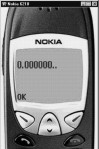Screen 10. 32

aaa.wmls

extern function abc()

{

var aa;

aa = String.format("%f..", 6 > 40);

}

The point to note here is that all the logical operators return a value of either true or false.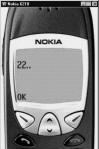Screen 10. 33

aaa.wmls

extern function abc()

{

var i,aa;

i = 12;

i += 10 ;

aa = String.format("%d..",i);

}

In the above example, we have an integer i which has been initialized to 12. The next statement is i +=10. This statement is equivalent to i = i+10. So, the statement i+=10 is a short form of the same statement. This proves that there is a lot of redundancy in these languages because there are multiple ways to do the same thing.

There is an increment operator ++, which is used to increase the value of a variable by 1.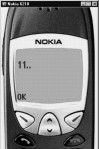Screen 10. 34

aaa.wmls

extern function abc()

{

var aa,i,j;

i = 10;

j = ++i;

aa = String.format("%d..", j);

}

The statement j = ++i, can be interpreted as follows: increment the value of i by 1 and then assign this value to j.

The statement j = i++, can be interpreted as follows: Assign the value of i to j and then increment the value of i by 1. In this case, when you display the value of j, it will be 10; but when you print the value of i, it will be 11.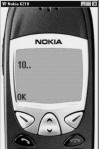Screen 10. 35

aaa.wmls

extern function abc()

{

var aa,i,j;

i = 10;

j = i++;

aa = String.format("%d..", j);

}

There is one construct that you need to avoid, and that is, using ++ operator in the numerator and the denominator of an expression. This is because the result of such an expression is unpredictable.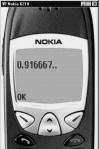Screen 10. 36

aaa.wmls

extern function abc()

{

var aa,i,j;

i = 10;

j = ++i/++i;

aa = String.format("%f..", j);

}

The question here is that, at the time of execution, which expression gets evaluated first; is it the numerator or is it the denominator? Let us assume that the initial value of i is 10.

Fortunately, the first thing that gets evaluated is the numerator. So, its value becomes 11. Now, because the value of i has become 11, the ++i in the denominator will make the value of the denominator 12. So, the resultant expression becomes 11/12. That is why the final answer is .9167.

Precedence of Operators

Whenever you have multiple operators on a single line, they are evaluated as per a fixed order of  precedence. This order is specified in the precedence table in the documentation.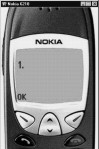Screen 10. 37

aaa.wmls

extern function abc()

{

var aa,a,b;

b = 6;

a = b = 7  != 8 ;

aa = String.format("%d.",b);

}

Between the = and !=, the != operator gets executed first, because it has a greater precedence than = operator.

In the expression b=7!=8, the statement 7!=8 is true, so the value of b becomes 1. The expression  a=b results in the assignment of the value 1 to a. So, when you print the value of a or b, the output will be 1.

Use of Brackets

When you use brackets, the order of evaluation gets changed because the expression within the brackets gets evaluated first. Thus, the value of b becomes 7, and since the expression 7 !=8 is true, the value of the variable a becomes 1.

Brackets are used to override the general rules of precedence. Whenever there are multiple operators in the same expression, you should use brackets to ensure the desired order of evaluation of the expressions..

Error Checking

WMLScript does a lot of error checking.

aaa.wmls

extern function abc()

{

pqr(12);

}

extern function pqr()

{

}

The first error check that it carries out is, to check the number of parameters passed to a function. It compares the number of parameters actually passed and the number of parameters expected.

If the function pqr expects one parameter and you call it with no parameters, it reports an error.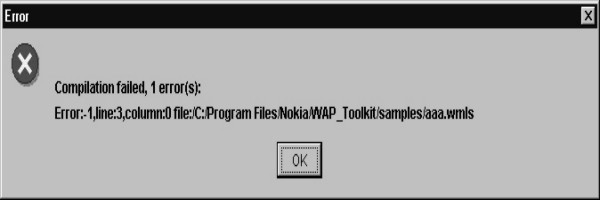Screen 10. 38

In the following example, we have a function called lang.maxInt which gives the value of the largest integer. Even when we add 1 to this value, it doesn't generate any error .

aaa.wmls

extern function abc()

{

var a = Lang.maxInt();

var b = a + 1;

var aa = String.format("%d..",b);